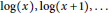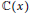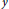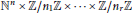Home

# Tannakian Categories With Semigroup Actions

## Abstract

A theorem of Ostrowski implies that$\log \left( x \right),\,\log \left( x+1 \right),\,.\,.\,.$ are algebraically independent over$\mathbb{C}\left( x \right)$ . More generally, for a linear differential or difference equation, it is an important problem to find all algebraic dependencies among a non-zero solution$y$ and particular transformations of$y$ , such as derivatives of$y$ with respect to parameters, shifts of the arguments, rescaling, etc. In this paper, we develop a theory of Tannakian categories with semigroup actions, which will be used to attack such questions in full generality, as each linear differential equation gives rise to a Tannakian category. Deligne studied actions of braid groups on categories and obtained a finite collection of axioms that characterizes such actions to apply them to various geometric constructions. In this paper, we find a finite set of axioms that characterizes actions of semigroups that are finite free products of semigroups of the form${{\mathbb{N}}^{n}}\,\times \,\mathbb{Z}/{{n}_{1}}\mathbb{Z}\,\times \,.\,.\,.\,\mathbb{Z}/{{n}_{r}}\mathbb{Z}$ on Tannakian categories. This is the class of semigroups that appear in many applications.

## References

Hide All
MathJax
MathJax is a JavaScript display engine for mathematics. For more information see http://www.mathjax.org.

# Tannakian Categories With Semigroup Actions

## Metrics

### Full text viewsFull text views reflects the number of PDF downloads, PDFs sent to Google Drive, Dropbox and Kindle and HTML full text views.

Total number of HTML views: 0
Total number of PDF views: 0 *Loading metrics...

### Abstract viewsAbstract views reflect the number of visits to the article landing page.

Total abstract views: 0 *Loading metrics...

* Views captured on Cambridge Core between <date>. This data will be updated every 24 hours.

Usage data cannot currently be displayed.